Add & Subtract Rational Expressions MathBitsNotebook.com Terms of Use   Contact Person: Donna Roberts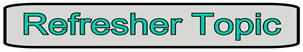This page will only be working with "simple" rational expressions which contain
numerical denominators, or simple monomial denominators with a variable.

 Adding (or subtracting) rational expressions (and other algebraic fractions) requires a COMMON DENOMINATOR. You need a common denominator to add numerical fractions, and you need a common denominator to add algebraic fractions.Steps to Adding (or subtracting) Rational Expressions:
Your teacher may, or may not, ask you to state the domains of rational expressions.

 Numerical Denominators: 1. Choose the least common denominator (LCD), which is the smallest value possible that all denominators can divide into without a remainder. 1. Given: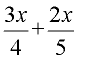The Least Common Denominator (LCD) is 20. Domain: All real numbers (no zero demonimator problems.) 2. Adjust each fraction to contain the new LCD. If any denominator is already the LCD, leave the numerator alone. If any denominator is not the LCD, multiply that numerator and denominator by the SAME value that is used to create the LCD. 2. To create the LCD of 20, the first term gets multiplied top and bottom by 5, and the second term gets multiplied top and bottom by 4.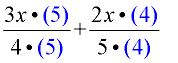3. Now that the denominators are the same, combine the problem into one fraction, and combine the terms in the numerator. Check to see if the final answer can be reduced further. 3.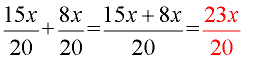Ans. Domain: All real numbers. This final answer cannot be reduced further. Variable Denominators: 4. When one or more of the denominators contains a variable, the same process (described above) is used. When the denominator contains a variable, the LCD will need to contain the largest power of that variable present. Start by finding the LCD. 4. Given: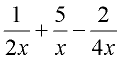The Least Common Denominator (LCD) is 4x. The largest power of x in this problem is 1, so the LCD contains x to the first power, multipied by the common denominator for 2 and 4 (which is 4). Domain: x ≠0 5. Adjust each fraction to contain the LCD. Be sure to adjust the numerator by the SAME value used to adjust its denominator. 5. Multiply each denominator (and its corresponding numerator) by a value what will create the LCD of 4x.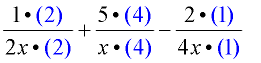It is not necessary to show the multiplication by (1). 6. Combine the problem into one fraction. Combine any similar terms in the numerator. 6.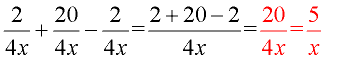Ans. Domain: x ≠ 0 This final answer was then reduced further.Examples:

 Example 1: (Use of parentheses.) Add: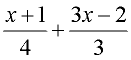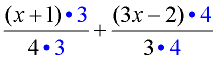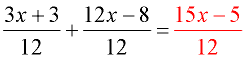Domain: All real numbers The LCD in this problem is 12. When adjusting for the LCD, it is necessary to multiply BOTH the numerator and denominator in each fraction by the numerical values of 3, and then 4.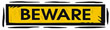The ENTIRE numerator, in each case, must be multiplied, as is shown by the use of parentheses. Be sure to distribute across the parentheses. Example 2: (Subtracting a quantity.) Subtract: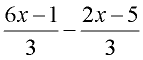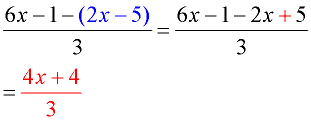Domain: All real numbers At first glance, this problem looks easy because it already has a common denominator. But be careful!!! A potential "problem spot" in this example is the subtraction of the second numerator.You must subtract the ENTIRE second numerator, not just the first term. Use parentheses around the numerator being subtracted!! When you distribute the negative sign across the parentheses you will be subtracting both terms in the second numerator. Example 3: (Variable denominators.)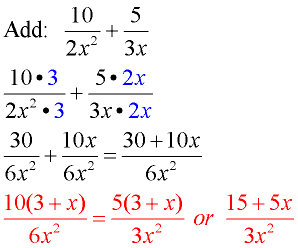Domain: x ≠ 0 The LCD in this problem is 6x2. The largest power of x is 2 and the common denominator for 2 and 3 is 6. Be careful to combine only "like" terms (30 and 10x are not "like" terms). When finished, look for common factors in the numerator to see if the answer can be reduced further. This fraction can be reduced further.